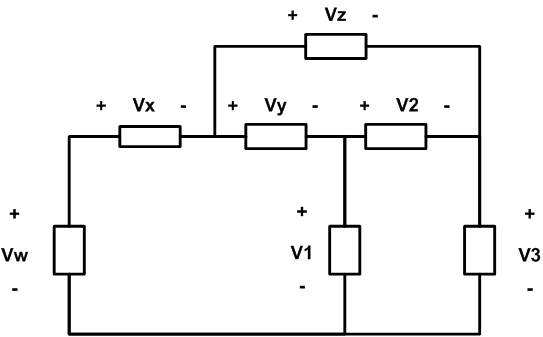# Calculate the voltages of the circuit.

• Engineering
jackashflash

## Homework StatementConsider the circuit above. Calculate V1, V2, and V3 when Vw = -9.8 V, Vx = -4.1 V, Vy = 2.1 V, and Vz = 1.9 V.

sum voltages = 0

## The Attempt at a Solution

None.

Where should I start on this problem, I really am at a loss?

Last edited:

Mentor

## Homework StatementConsider the circuit above. Calculate V1, V2, and V3 when Vw = -9.8 V, Vx = -4.1 V, Vy = 2.1 V, and Vz = 1.9 V.

sum voltages = 0

## The Attempt at a Solution

None.

Where should I start on this problem, I really am at a loss?

What circuit laws are you familiar with? Ever heard of KVL?

Start by penciling in the known values on your diagram...